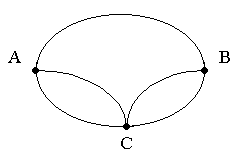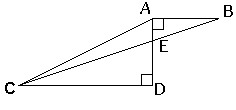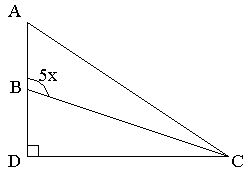UPSC  >  Problem Solving Practice Quiz - 1

# Problem Solving Practice Quiz - 1 - UPSC

Test Description

## 10 Questions MCQ Test UPSC Prelims Paper 2 CSAT - Quant, Verbal & Decision Making - Problem Solving Practice Quiz - 1

Problem Solving Practice Quiz - 1 for UPSC 2023 is part of UPSC Prelims Paper 2 CSAT - Quant, Verbal & Decision Making preparation. The Problem Solving Practice Quiz - 1 questions and answers have been prepared according to the UPSC exam syllabus.The Problem Solving Practice Quiz - 1 MCQs are made for UPSC 2023 Exam. Find important definitions, questions, notes, meanings, examples, exercises, MCQs and online tests for Problem Solving Practice Quiz - 1 below.
Solutions of Problem Solving Practice Quiz - 1 questions in English are available as part of our UPSC Prelims Paper 2 CSAT - Quant, Verbal & Decision Making for UPSC & Problem Solving Practice Quiz - 1 solutions in Hindi for UPSC Prelims Paper 2 CSAT - Quant, Verbal & Decision Making course. Download more important topics, notes, lectures and mock test series for UPSC Exam by signing up for free. Attempt Problem Solving Practice Quiz - 1 | 10 questions in 20 minutes | Mock test for UPSC preparation | Free important questions MCQ to study UPSC Prelims Paper 2 CSAT - Quant, Verbal & Decision Making for UPSC Exam | Download free PDF with solutions
 1 Crore+ students have signed up on EduRev. Have you?
Problem Solving Practice Quiz - 1 - Question 1

### Of the following, which is greater than 1 / 2?

Detailed Solution for Problem Solving Practice Quiz - 1 - Question 1

2 / 5 = 0.4
4 / 7 = 0.571
4 / 9 = 0.444
5 / 11 = 0.45
6 / 13 = 0.46
and,
1 / 2 = 0.5
So, only 4 / 7 > 1 / 2

Problem Solving Practice Quiz - 1 - Question 2

### If an object travels at five feet per second, how many feet does it travel in one hour?

Detailed Solution for Problem Solving Practice Quiz - 1 - Question 2

If an object travels at 5 feet per second it covers 5 x 60 feet in one minute, and 5 x 60 x 60 feet in one hour. Answer = 18000 (E)

Problem Solving Practice Quiz - 1 - Question 3

### What is the average (arithmetic mean) of all the multiples of ten from 10 to 190 inclusive?

Detailed Solution for Problem Solving Practice Quiz - 1 - Question 3

Method 2:

Take the average of the outermost two numbers. This will be the same as the average of all 19 numbers, because the numbers in between are evenly spaced out, in a symmetrical pattern.

(10 + 20 + ... + 180 + 190) / 19

= 10 + 190 / 2

= 200 / 2

= 100

Problem Solving Practice Quiz - 1 - Question 4

A cubical block of metal weighs 6 pounds. How much will another cube of the same metal weigh if its sides are twice as long?

Detailed Solution for Problem Solving Practice Quiz - 1 - Question 4

If you double the sides of a cube, the ratio of the surface areas of the old and new cubes will be 1 : 4. The ratio of the volumes of the old and new cubes will be 1 : 8.
Weight is proportional to volume. So, If the first weighs 6 pounds, the second weighs 6 x 8 pounds = 48.

Problem Solving Practice Quiz - 1 - Question 5

In a class of 78 students 41 are taking French, 22 are taking German. Of the students taking French or German, 9 are taking both courses. How many students are not enrolled in either course?

Detailed Solution for Problem Solving Practice Quiz - 1 - Question 5

You could solve this by drawing a Venn diagram. A simpler way is to realize that you can subtract the number of students taking both languages from the numbers taking French to find the number taking only French. Likewise find those taking only German. Then we have:Total = only French + only German + both + neither 78 = (41 - 9) + (22 - 9) + 9 + neither.

Not enrolled students = 24

Problem Solving Practice Quiz - 1 - Question 6

230 + 230 + 230 + 230 =

Detailed Solution for Problem Solving Practice Quiz - 1 - Question 6

4 (230) = 22 ×230 = 232

Problem Solving Practice Quiz - 1 - Question 7Amy has to visit towns B and C in any order. The roads connecting these towns with her home are shown on the diagram. How many different routes can she take starting from A and returning to A, going through both B and C (but not more than once through each) and not travelling any road twice on the same trip?

Detailed Solution for Problem Solving Practice Quiz - 1 - Question 7

Amy can travel clockwise or anticlockwise on the diagram.
Clockwise, she has no choice of route from A to B, a choice of one out of two routes from B to C, and a choice of one out of two routes from C back to A. This gives four possible routes.
Similarly, anticlockwise she has four different routes.
Total routes = 8

Problem Solving Practice Quiz - 1 - Question 8In the figure above AD = 4, AB = 3 and CD = 9. What is the area of triangle AEC ?

Detailed Solution for Problem Solving Practice Quiz - 1 - Question 8

If we take AE as the base of triangle AEC, then the height is CD.
The height of the triangle is therefore, 9 (given).
To find the base we need to see that triangles AEB and CDE are similar. The ratio AB: CD, is therefore equal to the ratio AE: ED. The given information shows that the ratio is 3:9, or 1:3. Now dividing AD (4) in this ratio gives us AE as 1.
The area of AEC = ½ base x height
=1/2 x 9 = 4.5

Problem Solving Practice Quiz - 1 - Question 9Which of the following could be a value of x, in the diagram above?

Detailed Solution for Problem Solving Practice Quiz - 1 - Question 9

The marked angle, ABC must be more than 90 degrees because it is the external angle of triangle BDC, and must be equal to the sum of angles BDC (90) and DCB.
Also ABC is not a straight line and must be less than 180.
Therefore 90 < 5x < 180
The only value of x which satisfies this relation is 20.

Problem Solving Practice Quiz - 1 - Question 10

Helpers are needed to prepare for the fete. Each helper can make either 2 large cakes per hour, or 35 small cakes per hour. The kitchen is available for 3 hours and 20 large cakes and 700 small cakes are needed. How many helpers are required?

Detailed Solution for Problem Solving Practice Quiz - 1 - Question 10

20 large cakes will require the equivalent of 10 helpers working for one hour. 700 small cakes will require the equivalent of 20 helpers working for one hour. This means if only one hour were available we would need 30 helpers. But since three hours are available we can use 10 helpers.

## UPSC Prelims Paper 2 CSAT - Quant, Verbal & Decision Making

67 videos|50 docs|151 tests
Information about Problem Solving Practice Quiz - 1 Page
In this test you can find the Exam questions for Problem Solving Practice Quiz - 1 solved & explained in the simplest way possible. Besides giving Questions and answers for Problem Solving Practice Quiz - 1, EduRev gives you an ample number of Online tests for practice

## UPSC Prelims Paper 2 CSAT - Quant, Verbal & Decision Making

67 videos|50 docs|151 tests

### How to Prepare for UPSC

Read our guide to prepare for UPSC which is created by Toppers & the best Teachers(Scan QR code)